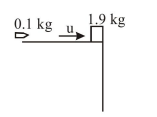# A block of mass 1.9kg is at rest at the edge of a table,

Question:

A block of mass $1.9 \mathrm{~kg}$ is at rest at the edge of a table, of height $1 \mathrm{~m}$. A bullet of mass $0.1 \mathrm{~kg}$ collides with the block and sticks to it. If the velocity of the bullet is $20 \mathrm{~m} / \mathrm{s}$ in the horizontal direction just before the collision then the kinetic energy just before the combined system strikes the floor, is [Take $g=10 \mathrm{~m} / \mathrm{s}^{2}$. Assume there is no rotational motion and loss of energy after the collision is negligable.]

1. $21 \mathrm{~J}$

2.  $23 \mathrm{~J}$

3. $19 \mathrm{~J}$

4. $20 \mathrm{~J}$

Correct Option: 1

Solution:$\mathrm{p}_{\mathrm{i}}=\mathrm{p}_{\mathrm{f}} \Rightarrow 0.1 \times 20=2 \mathrm{v}$

$\therefore \mathrm{V}=1 \mathrm{~m} / \mathrm{s}$

$\mathrm{KE}_{\mathrm{f}}=\mathrm{mgh}+\frac{1}{2} \mathrm{mv}^{2}=213$